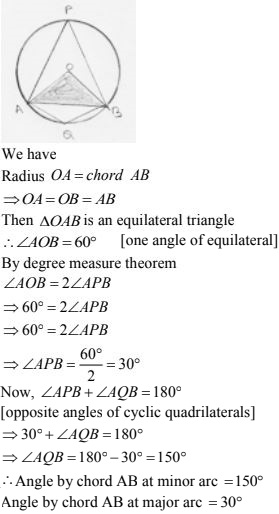# Chapter 16 Circles RD Sharma Solutions Exercise 16.4 Class 9 Maths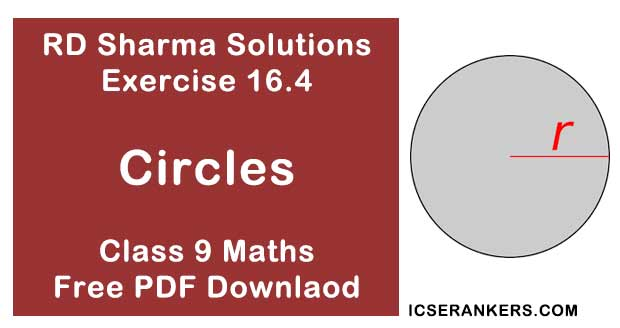Chapter Name RD Sharma Chapter 16 Circles Exercise 16.4 Book Name RD Sharma Mathematics for Class 10 Other Exercises Exercise 16.1Exercise 16.2Exercise 16.3Exercise 16.5 Related Study NCERT Solutions for Class 10 Maths

### Exercise 16.4 Solutions

1. In the below fig. O is the centre of the circle. If ∠APB = 50°, find ∠AOB and ∠OAB.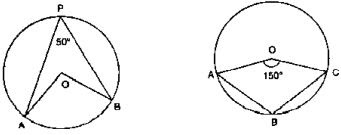Solution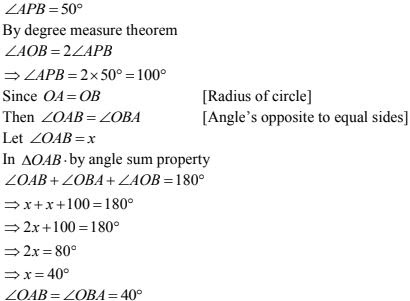2. In the fig below, it is given that O is the centre of the circle and ∠AOC = 150°. Find ∠ABC.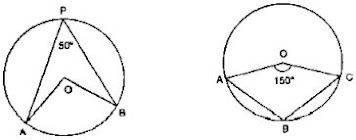Solution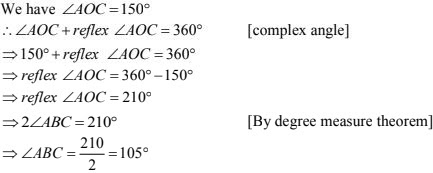3. In the below fig. O is the centre of the circle. Find ∠BAC.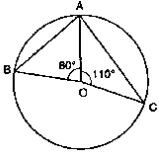Solution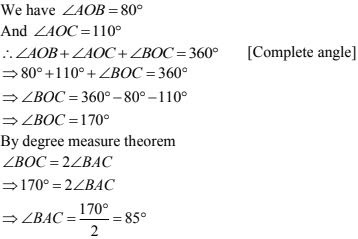4. If O is the centre of the circle, find the value of x in each of the following figures :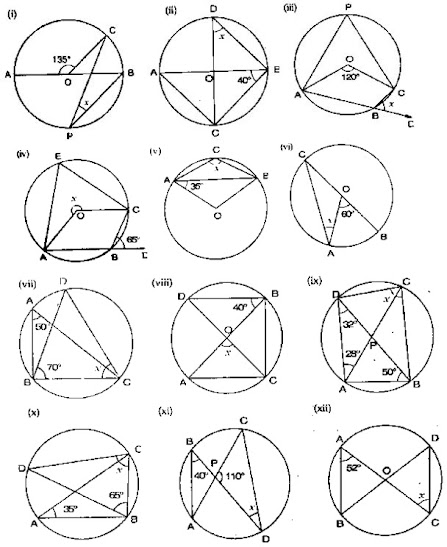Solution

(i) ∠AOC = 135°
∴ ∠AOC + ∠BOC = 180°  [Linear pair of angles]
⇒ 135° + ∠BOC = 180°
⇒ ∠BOC = 180° - 135° = 45°
By degree measures theorem
∠BOC = 2∠CDB
⇒ 45° = 2x
⇒x = 45/2 = 22.5°

(ii) We have
∠ABC = 40°
∠ACB = 90°  [Angle in semicircle]
In ΔABC, by angle sum property
∠CAB + ∠ACB + ∠ABC = 180°
⇒ ∠CAB + 90° +40° = 180°
⇒∠CAB = 180° - 130°
⇒ ∠CAB = 50°
Now,
∠COB = ∠CAB  [Angle is same segment]
⇒ x = 50°

(iii) We have
∠AOC = 120°
By degree measure theorem
∠AOC = 2∠APC
⇒ 120° = 2∠APC
⇒ ∠APC = 120°/2 = 60°
∠APC + ∠ABC = 180°  [Opposite angles of cyclic quadrilateral]
⇒ 60° + ∠ABC = 180°
⇒ ∠ABC = 180° - 60°
⇒ ∠ABC = 120°
∴ ∠ABC + ∠DBC = 180°  [Linear pair of angles]
⇒ 120° + x = 180°
⇒ x = 180° - 120° = 60°

(iv) We have
∠CBD = 65°
∴ ∠ABC + ∠CBD = 180° [Linear pair of angles]
⇒ ∠ABC + 65° = 180°
⇒ ∠ABC = 180° - 65° = 115°
∴ Reflex ∠AOC = 2∠ABC  [By degree measure theorem]
⇒ x = 2 × 115°
⇒ x = 230°

(v) We have
∠OAB = 35°
Then, ∠OBA = ∠OAB = 35° [Angles opposite to equal radii]
In ΔAOB, by angle sum property
∠AOB + ∠OAB + ∠OBA = 180°
⇒ ∠AOB + 35° + 35° = 180°
⇒ ∠AOB = 180° - 70° = 110°
∴∠AOB + reflex ∠AOB = 360°  [complete angle]
⇒ 110° + reflex ∠AOB = 360°
⇒ reflex ∠AOB = 360° - 110° = 250°
By degree measure theorem reflex ∠AOB = 2∠ACB
⇒ 250° = 2x
⇒ x = 250°/2 = 125°

(vi) We have
∠AOB = 60°
By degree measure theorem
∠AOB = 2∠ACB
⇒ 60° = 2∠ACB
⇒ ∠ACB =60°/2 = 30°  [Angles opposite to equal radii]
⇒ x = 30°

(vii) We have
∠BAC = 50°
And ∠DBC = 70°
∴ ∠BDC = ∠BAC = 50°  [Angle in same segment]
In ΔBDC, by angles sum property
∠BDC + ∠BCD + ∠DBC = 180°
⇒ 50° + x + 70° = 180°
⇒ x = 180° - 70° - 50° = 60°

(viii) We have
∠DBO = 40°
∠DBC = 90°  [Angle in semi circle]
⇒ ∠DBO + ∠OBC = 90°
⇒ 40° + ∠OBC = 90°
⇒ ∠OBC = 90° - 40° = 50°
By degree measure theorem
∠AOC = 2∠OBC
⇒ x = 2 × 50° = 100°

(ix) In ΔDAB, by angle sum property
∠ADB + ∠DAB + ∠ABD = 180°
⇒ 32° + ∠DAB + 50° = 180°
⇒ ∠OAB = 180° - 32° - 50°
⇒ ∠DAB = 95°
Now, ∠OAB + ∠DCB = 180°  [Opposite angles of cyclic quadrilateral]
⇒ 98° + x = 180°
⇒ x = 180° - 98° = 82°

(x) we have
∠BAC = 35°
∠BAC = ∠BAC = 35°  [Angle in same segment]
In ΔBCD by angle sum property
∠BDC + ∠BCD + ∠DBC = 180°
⇒ 35° + x + 65° = 180°
⇒ x = 180° - 35° - 66° = 80°

(xi) We have
∠ABD = 40°
∴ ∠ACD = ∠ABD = 40°  [Angle in same segment]
In ΔPCD, by angle sum property
∠PCD + ∠CPO + ∠PDC = 180°
⇒ 40° + 110° + x = 180°
⇒ x = 180° - 150°
⇒ x = 30°

(xii) Given that ∠BAC = 52°
Then, ∠BDC = ∠BAC = 52° [Angle in same segment]
Since  OD = OC
Then, OD = OC
Then, ∠ODC = ∠OCD
⇒ x = 50°

5. O is the circumcenter of the triangle ABC and OD is perpendicular on BC. Prove that ∠BOD = ∠A.

Solution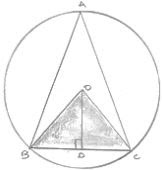Given O is the circumcenter  of  ΔABC and OD ⊥ BC
To prove ∠BOD = 2∠A
Proof :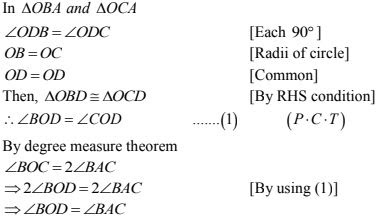6. In the fig. below, O is the centre of the circle, BO is the bisector of ∠ABC. Show that AB = AC.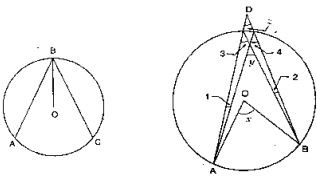Solution
Given, BO is the bisector of ∠ABC
To prove: AB = BC
Proof:
Since, BO is the bisector of ∠ABC
Then, ∠ABO = ∠DAB ...(2) [Opposite angles to equal sides]
Since OB = OC  [Radius of circle]
The ∠CBO = ∠OCB  ...(3)  [Opposite angles to equal sides]
Compare equation (1), (2)  and (3)
∠OAB = ∠OCB  ...(4)
In ∠OAB = ∠OCB  [From (4)]
∠OBA = ∠OBC  [Given]
OB = OB   [Common]
Then, ΔOAB ≅ OCB  [By AAS condition]
∴ AB = BC [c.p.c.t]

7. In the below fig. O is the centre of the circle, prove that ∠x = ∠y + ∠z.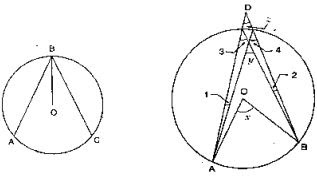Solution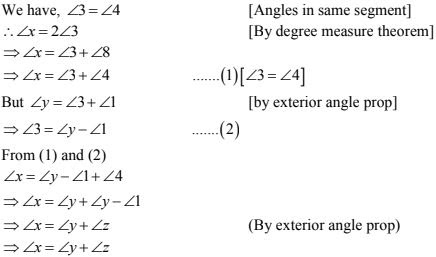8. In the below fig. O and O' are centres of two circles intersecting at B and C, ACD is a straight line, find x.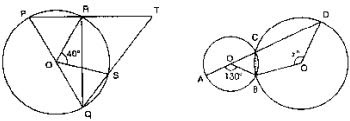Solution
By degree measure theorem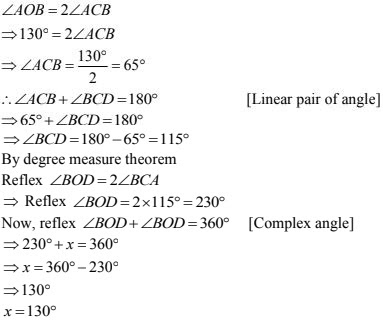9. In the below fig. O is the centre and PQ is a diameter. If ∠ROS = 40° , find ∠RTS.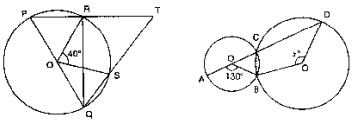Solution
Since PQ is diameter
Then, ∠PRO = 90°  [Angle in semi-circle]
∴ ∠PRQ + ∠TRQ = 180°  [Linear pair of angle]
⇒ ∠90° + ∠TRQ = 180°
⇒ ∠TRQ = 180° - 90° = 90°
By degree measure theorem
∠ROS  = 2∠RQS
⇒ 40° = 2∠RQS
⇒ ∠RQS = 40°/2 = 20°
In ΔRQT, By angle sum property
∠RQT + ∠QRT + ∠RTS = 180°
⇒ 20° + 90° + ∠R + ∠S = 180°
⇒ ∠RTS = 180° - 20° - 90° = 70°

10. In the below fig. if ∠ACB = 40°, ∠DPB = 120° , find ∠CBD.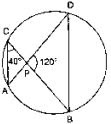Solution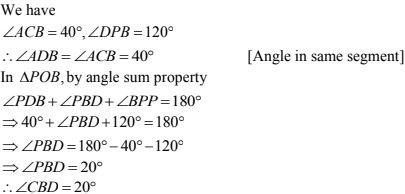11. A chord of a circle is equal to the radius of the circle. Find the angle subtended by the chord at a point on the minor arc and also at a point on the major arc.
Solution